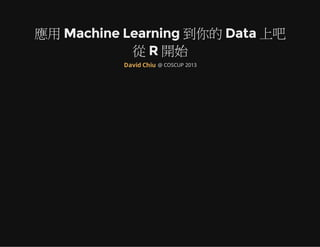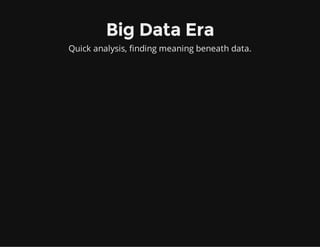Successfully reported this slideshow.

# Machine Learning With R×

1 of 41
1 of 41

# Machine Learning With R

Machine Learning With R @ COSCUP 2013

Machine Learning With R @ COSCUP 2013

### Machine Learning With R

1. 1. 應用 Machine Learning 到你的 Data 上吧 從 R 開始 @ COSCUP 2013David Chiu
2. 2. About Me Trend Micro Taiwan R User Group ywchiu-tw.appspot.com
3. 3. Big Data Era Quick analysis, finding meaning beneath data.
4. 4. Data Analysis 1. Preparing to run the Data (Munging) 2. Running the model (Analysis) 3. Interpreting the result
5. 5. Machine Learning Black-box, algorithmic approach to producing predictions or classifications from data A computer program is said to learn from experience E with respect to some task T and some performance measure P, if its performance on T, as measured by P, improves with experience E Tom Mitchell (1998)
6. 6. Using to do Machine Learning Using R
7. 7. Why Using R? 1. Statistic analysis on the fly 2. Mathematical function and graphic module embedded 3. FREE! & Open Source!
8. 8. Application of Machine Learning 1. Recommender systems 2. Pattern Recognition 3. Stock market analysis 4. Natural language processing 5. Information Retrieval
9. 9. Facial Recognition
10. 10. Topics of Machine Learning Supervised Learning Regression Classfication Unsupervised Learning Dimension Reduction Clustering
11. 11. Regression Predict one set of numbers given another set of numbers Given number of friends x, predict how many goods I will receive on each facebook posts
12. 12. Scatter Plot dataset <- read.csv('fbgood.txt',head=TRUE, sep='t', row.names=1) x = dataset\$friends y = dataset\$getgoods plot(x,y)
13. 13. Linear Fit fit <- lm(y ~ x); abline(fit, col = 'red', lwd=3)
14. 14. 2nd order polynomial fit plot(x,y) polyfit2 <- lm(y ~ poly(x, 2)); lines(sort(x), polyfit2\$fit[order(x)], col = 2, lwd = 3)
15. 15. 3rd order polynomial fit plot(x,y) polyfit3 <- lm(y ~ poly(x, 3)); lines(sort(x), polyfit3\$fit[order(x)], col = 2, lwd = 3)
16. 16. Other Regression Packages MASS rlm - Robust Regression GLM - Generalized linear Models GAM - Generalized Additive Models
17. 17. Classfication Identifying to which of a set of categories a new observation belongs, on the basis of a training set of data Given features of bank costumer, predict whether the client will subscribe a term deposit
18. 18. Data Description Features: age,job,marital,education,default,balance,housing,loan,contact Labels: Customers subscribe a term deposit (Yes/No)
19. 19. Classify Data With LibSVM library(e1071) dataset <- read.csv('bank.csv',head=TRUE, sep=';') dati = split.data(dataset, p = 0.7) train = dati\$train test = dati\$test model <- svm(y~., data = train, probability = TRUE) pred <- predict(model, test[,1:(dim(test)[]-1)], probability = TRUE)
20. 20. Verify the predictions table(pred,test[,dim(test)]) pred no yes no 1183 99 yes 27 47
21. 21. Using ROC for assessment library(ROCR) pred.prob <- attr(pred, "probabilities") pred.to.roc <- pred.prob[, 2] pred.rocr <- prediction(pred.to.roc, as.factor(test[,(dim(test)[])])) perf.rocr <- performance(pred.rocr, measure = "auc", x.measure = "cutoff") perf.tpr.rocr <- performance(pred.rocr, "tpr","fpr") plot(perf.tpr.rocr, colorize=T, main=paste("AUC:",(perf.rocr@y.values)))
22. 22. Then, get your thesis
23. 23. Support Vector Machines and Kernel Methods e1071 - LIBSVM kernlab - SVM, RVM and other kernel learning algorithms klaR - SVMlight rdetools - Model selection and prediction
24. 24. Dimension Reduction Seeks linear combinations of the columns of X with maximalvariance Calculate a new index to measure economy index of each Taiwan city/county
25. 25. Economic Index of Taiwan County 縣市 營利事業銷售額 經濟發展支出佔歲出比例 得收入者平均每人可支配所得 2012年《天下雜誌》幸福城市大調查 - 第505期
26. 26. Component Bar Plot dataset <- read.csv('eco_index.csv',head=TRUE, sep=',', row.names=1) pc.cr <- princomp(dataset, cor = TRUE) plot(pc.cr)
27. 27. Component Line Plot screeplot(pc.cr, type="lines") abline(h=1, lty=3)
28. 28. PCA biplot biplot(pc.cr)
29. 29. PCA barplot barplot(sort(-pc.cr\$scores[,1], TRUE))
30. 30. Other Dimension Reduction Packages kpca - Kernel PCA cmdscale - Multi Dimension Scaling SVD - Singular Value Decomposition fastICA - Independent Component Analysis
31. 31. Clustering Birds of a feather flock together Segment customers based on existing features
32. 32. Customer Segmentation Clustering by 4 features Visit Time Average Expense Loyalty Days Age
33. 33. Determing Clusters mydata <- read.csv('costumer_segment.txt',head=TRUE, sep='t') mydata <- scale(mydata) d <- dist(mydata, method = "euclidean") fit <- hclust(d, method="ward") plot(fit)
34. 34. Cutting trees k1 = 4 groups <- cutree(fit, k=k1) rect.hclust(fit, k=k1, border="red")
35. 35. Kmeans Clustering fit <- kmeans(mydata, k1) plot(mydata, col = fit\$cluster)
36. 36. Principal Component Plot library(cluster) clusplot(mydata, fit\$cluster, color=TRUE, shade=TRUE, lines=0)
37. 37. Other Clustering Packages kernlab - Spectral Clustering specc - Spectral Clustering fpc - DBSCAN
38. 38. Machine Learning Dignostic 1. Get more training examples 2. Try smaller sets of features 3. Try getting additional features 4. Try adding polynomial features 5. Try parameter increasing/decreasing
39. 39. Overfitting Trainging error to be low, test error to be highe. g. θJtraining θJtest
40. 40. Use For Data Analysis
41. 41. THANK YOU Please Come and Visit Taiwan R User Group